# Hydrolysis, Salts and Types

Table of Content:

## What is Hydrolysis?

Salts are strong electrolytes and ionize fully to form ions. These ions may react with water and form their conjugate pairs. Depending on the strength of the pairs, the cation or anion may undergo hydrolysis resulting in an increase in the hydrogen or hydroxide ion concentrations, making the solution acidic or basic or neutral. So, it is the reverse of neutralization reaction.

Salt BA ionizes as, $BA\rightleftharpoons B^{+} + A^{-}$

Cation hydrolysis $B^{+}+2H_2O\rightleftharpoons BOH + H_{3}O^{+}$

Anion hydrolysis $A^{-}+H_2O\rightleftharpoons HA + OH^{-}$

Hydrolysis of salt $Salt + Water\rightleftharpoons Acid + Base$

## Factors Determining the Extend of Hydrolysis

### Complete Hydrolysis

If the cation/anion and water are stronger than their conjugate pairs B+ is more acidic than the conjugate hydronium ion and water is more basic than conjugate BOH A is more basic than the conjugate hydroxide ion and water is more acidic than conjugate HA.

Example: ion that undergoes complete hydrolysis.

• $PH_{4}^{+} + H_2O\rightarrow PH_3 + H_3O^{+}$ form acid solution.
• NH2, Hform a basic solution.
• $H^{-} + H_2O\rightarrow H_2 + OH^{-}$ form basic solution.

### No Hydrolysis

If the cation/anion and water are weaker than their conjugate pairs B+ is less acidic than the conjugate hydronium ion and conjugate BOH is more basic than water A is less basic than the conjugate hydroxide ion and water is less acidic than conjugate HA.

Example: ion that does not undergo complete hydrolysis.

• Cations of strong bases: Alkali and alkaline metal ions.
• Anions of strong acids: Chloride, nitrate, sulphate, phosphate chlorate ions.

⇒ Na+ + H2O NaOH + H3O

⇒ Cl + H2O HCl + OH

### Limited Hydrolysis

When the cations or anions are not so strong compared to their conjugate pairs, hydrolysis relative to strength will take place and accordingly the solution may be acidic or basic.

Ions undergoing limited hydrolysis: Cations of weak bases result in the acidic solution. Anions of weak acids yield basic solutions.

NH4+, Al3+ CH3COO, CN. C2O42-, PO43-

⇒ $NH_{4}^{+}+H_2O\rightleftharpoons NH_4OH+H_3O^{+}$

⇒ $CH_3COO^{-}+H_2O\rightleftharpoons CH_3COOH + OH^{-}$

## What are Salts?

Acid and base react to form the salt. Salts are of four types.

• Salt of the strong acid and strong base – NaCl
• Salt of the strong acid and weak base – NH4Cl, FeCl3, CuCl2, AlCl3, CuSO4
• Salt of the weak acid and strong base – CH3COONa, NaCN, NaHCO3, Na2CO3,
• Salt of the weak acid and weak base – CH3COONH4, (NH4)2CO3, NH4HCO3

### pH Aqueous Solutions of Salt of a Strong Acid and Strong Base

Anions and cations of the strong acid and bases do not undergo hydrolysis with water and so the

the solution will be neutral, with pH = 7.

pH aqueous solutions of Salt of the strong acid and weak baseNH4Cl, FeCl3, CuCl2, AlCl3, CuSO4

Only the cation of the weak base undergoes hydrolysis to make the solution acidic.

Say, ‘h’ be the degree of hydrolysis-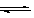NH4Cl NH4+ + ClNH4+ + H2O NH4OH + H+

At start, Concentration, mole/l C 0 0

At equilibrium C(1-h) Ch Ch

$Hydrolysis\; constant = K_h = \frac{[NH_4OH][H^{+}]}{[NH_{4}^{+}]} \frac{Ch+Ch}{C(1-h)}=\frac{ch^{2}}{(1-h)} = ch^{2}$; h is small, so (1-h)=1

$h = \sqrt{\frac{Kh}{c}} \; ;[H^{+}] = ch = \sqrt{cKh}$

For the equilibrium, $NH_4OH \rightleftharpoons NH_{4}^{+} + OH^{-}\;\;\;Equilibrium\; constant\; of\; the\; base = Kb = \frac{[NH_{4}^{+}][OH^{-}]}{[NH_4OH]}$

For the equilibrium, $H_2O \rightleftharpoons H^{+} + OH^{-}\;\;\;Ionic\; product\; of\; water\; = Kw = [H^{+}][OH^{-}]=10^{-14}$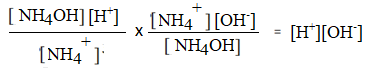Kh x Kb = Kw ; $Kh= \frac{Kw}{Kb}$

$So\; [H^{+}]= ch=\sqrt{cKh} = \sqrt{c \frac {Kw}{Kb}} = – \frac{1}{2}[\log Kw – \log Kb + \log c]$

pH aqueous solutions of Salt of strong acid and weak base $pH = \frac{1}{2}[pKw-pKb-\log c]$

c) pH aqueous solutions of Salt of weak acid and strong base-CH3COONa, NaCN, NaHCO3, Na2CO3,

$CH_3COONa\rightleftharpoons Na^{-} + CH_3COO^{-}$

$CH_3COO^{-} + H_2O\rightleftharpoons CH_3COOH + OH^{-}$

At start, Concentration, mole/l C 0 0

At equilibrium C(1-h) Ch Ch

$Hydrolysis\; constant = Kh = \frac{[CH_3COOH][OH^{-}]}{[CH_3COO^{-}]} = \frac{(ch+ch)}{c(1+h)} = \frac{ch^{2}}{(1-h)} = CH^{2}$; h is small, so (1-h)=1

$h = \sqrt{\frac{Kh}{c}}; [OH^{-}] = ch = \sqrt{cKh}$

For the equilibrium, $CH_3COOH\rightleftharpoons CH_3COO^{-} + H^{+}\;Equlibrium constant of acid=Ka=\frac{[CH_3COO^{-}][H^{+}]}{CH_3COOH}$

For the equilibrium, $H_2O\rightleftharpoonsH^{+} + OH^{-} \;Ionic\;product\;of \;water = Kw=[H^{+}][OH^{-}]=10^{-14}$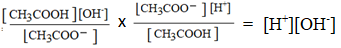Kh x Ka = Kw ; $Kh = \frac{Kw}{Ka}$

So, $[0H^{-}] = ch = \sqrt{cKh} = \sqrt {c\frac{Kw}{Ka}}\;and\;=-\log \sqrt{c\frac{Kw}{Ka}} = -\frac{1}{2}[\log Kw-\log Ka +\log c]\;\;\; pOH = \frac{1}{2}[pKw – pKa – \log c]$

$pH = 14-pOH = 14-\frac{1}{2}[pKw – pKa – \log c] = 14+\frac{1}{2}[-pKw + pKa+ \log c]$

$pH = \frac{1}{2}[28-pKw+pKa+\log c] = \frac{1}{2}[2pKw – pKw + pKa + \log c] = \frac{1}{2}[pKw +pKa +\log c]$

$pH \;laqueous\;solution \;of\;salt\;of\;weak\;acid\;and\;strong\;base pH = \frac{1}{2}[pKw+pKb+\log c]$

d) pH aqueous solutions of Salt of weak acid and weak base: CH3COONH4, (NH4)2CO3, NH4HCO3

$CH_3COO NH_4 \rightleftharpoons NH_{4}^{+} + CH_3COO^{-}$

$CH_3COO^{-}+NH_{4}^{+}+H_2O \rightleftharpoons CH_3COOH + NH_4OH$

At start, Concentration, mole/l C C 0 0

At equilibrium C(1-h) C(1-h) Ch Ch

$Hydrolysis\;constant = Kh = \frac{[CH_3COOH][NH_4OH]}{[CH_3COO^{-}][NH_{4}^{+}]} = \frac{(ch+ch)}{c(1-h)c(1-h)} = \frac{h^{2}}{((1-h)^{2})} ;$

$Kh = \frac{h^{2}}{1-h};\frac{h}{1-h} = \sqrt{Kh}$

For the equilibrium, $CH_3COOH \rightleftharpoons CH_3COO^{-} + H^{+}$

$Equilibrium \;constant\;of\;acid = Ka = \frac{[CH_3COO^{-}][H^{+}]}{[CH_3COOH]}[H^{+}] = Ka\times \frac{[CH_3COOH]}{[CH_COO^{-}]}$

$[H^{+}] = Ka\frac{ch}{c(1-h)} = Ka\frac{h}{1-h} = Ka\sqrt{kh}$

For the equilibrium, $NH_4OH\rightleftharpoons NH_{4}^{+} +OH^{-}\;\;Equilibrium\;constant\;of\;the\;base = Kb = \frac{[NH_{4}^{-}][OH^{-}]}{NH_4OH}$

For the equilibrium, $H_2O\rightleftharpoons H^{+} + OH^{-} \;\;Ionic\;product\;of\;water = Kw = [H^{+}][OH^{-}] = 10^{-14}$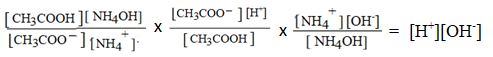Kh x Ka x Kb = Kw ; $Kh = \frac{Kw}{KaKb}$

$[H^{+}] = Ka\sqrt{Kh} = Ka\frac{Kw}{KaKb} = \sqrt{\frac{KwKa}{Kb}}$

$pH = -\log[H^{+}] = -\log[\sqrt{\frac{KwKa}{Kb}}] =-\frac{1}{2}[\log Kw+\log Ka – \log Kb]= \frac{1}{2}[pKw+pKa-pKb]$

pH aqueous solutions of Salt of weak acid and strong base $pH = \frac{1}{2}[pKw +pKb-pKb]$

e) pH of polyprotic acids and their salts:

1) pH of polyprotic acids:

Acids that can ionize to give two or more hydrogen ions are polyprotic acids.

Examples: Sulphuric, phosphoric, cabonic, oxalic acid are some.

These acid ionize in steps. But the ionization may stop with the first ionization unless it is required

due to common ions produced in the earlier steps.

Orthophosphoric acid can ionize in three steps two yield three hydrogen ions as follows.

$H_3PO_4\rightleftharpoons H^{+}+H_2PO_{4}^{-}\;\;\;\;Ka1 = \frac{[H^{+}][H_2PO]_{4}^{1}}{[H_3PO_4]}$

$H_2PO_{4}^{-}\rightleftharpoons H^{+}+HPO_{4}^{-}\;\;\;\;Ka2 = \frac{[H^{+}][HPO_{4}^{2-}]}{[H_2PO_{4}^{-}]}$

$H_2PO_{4}^{-}\rightleftharpoons H^{+}PO_{4}^{-}\;\;\;\;Ka3 = \frac{[H^{+}][HPO_{4}^{3-}]}{[HPO_{4}^{2-}]}$

For all acids, Ka1>> Ka2 >>Ka3….

So, pH of the acids are calculated on the first ionization constant only.

For weak acids, $Ka1 = \frac{[H^{+}][H_{2}PO_{4}^{3-}]}{[H_{3}PO_{4}]} = \frac{Ch-Ch}{C(1-h)} = \frac{ch^{2}}{(1-h)} ,h< < < 1,(1-\alpha) = 1$

.·. Ka1 = Ch2 $h=\sqrt{\frac{Ka1}{c}}\;\;\;\;[H^{+}]\;of\;ion=Ch\; and$

$pH = -\log\sqrt{CKa1} = \frac{1}{2}(-\log Ka1 – \log C);\;\;\;\; pH = \frac{1}{2}(pKal-\log C)$

b) pH of salts of polyprotic acids:K3PO4, Na2CO3, FeCl3, (NH4)C2O4

Salts will be completely ionized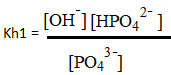Phosphate hydrolyzes as follows: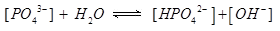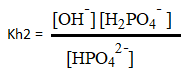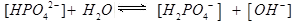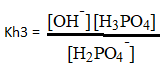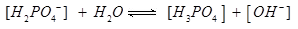On hydrolysis, [PO43-] + H2O [HPO42-] + [OH]

At equilibrium, moles/l C(1-h) Ch Ch

$Kh1 = \frac{(Ch+Ch)}{C(1-h)} = \frac{Ch^{2}}{(1-h)} ,h< < < 1, (1-h) = 1$

$Kh1 = Ch^{2} ; h= \sqrt {\frac{Kh1}{C}} ; [H^{+}] = \frac{Kw}{OH^{[-]}} = \frac{Kw}{\sqrt{CKh1}}$

$HPO_{4}^{-} \rightlefthrapoons H^{+} + PO_{4}^{-}$ $Ka3 = \frac{[H^{+}][PO_{4}^{3}]}{[HPO_{4}^{2-}]}$

$Kh1 \times Ka3 = \frac{[OH^{-}][HPO_{4}^{2-}]}{[PO_{4}^{3-}]} \frac{[H^{+}][PO_{4}^{3-}]}{[OH^{-}]} = [H^{+}][OH^{-}] = Kw$

Hence $Kh1 = \frac{Kw}{Ka3}$

$[H^{+}] = \frac{Kw}{\sqrt{CKh1}} = \sqrt{\frac{KwKa3}{C}} ; pH = -\log\sqrt{\frac{KwKa3}{C}} = -\frac{1}{2}(\log Kw+\log Ka3 – \log C)$

$pH = -\frac{1}{2}(pKw+pKa3+\log C)$

pH of salts of polyprotic acids,K3PO4 = $= – \frac{1}{2}(pKw+pKa3+\log C)$

### Hydrolysis of Amphoteric Anion

NaHCO3, NaHS

Amphoteric anions can ionize to give hydrogen ion and hydrolyze to give hydroxide ion.

⇒ Ionization $HCO_{3}^{-} + H_2O \rightleftharpoons CO_{3}^{2-} + H_3O^{+} ; with Ka1$

⇒ Hydrolysis $HCO_{3}^{-} + H_2O \rightleftharpoons H_2CO_3 + OH^{-} ; with Ka2$

$pH_{(HCO_{3-})} = \frac{pKa1+pKa2}{2}$